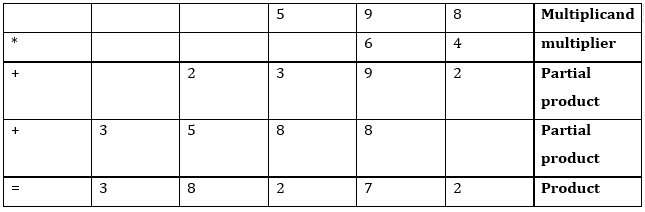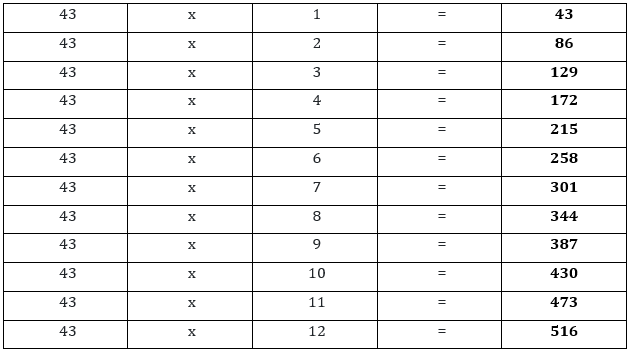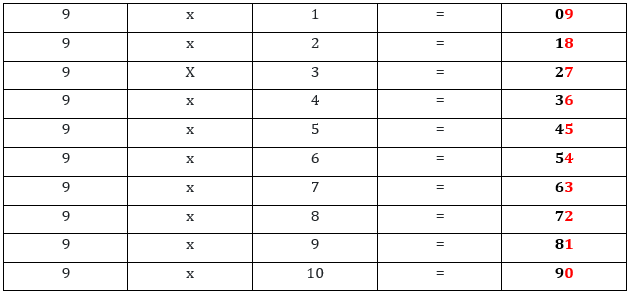# Introduction to multiplication and multiplication tables

In an earlier era, it is a big problem to add larger numbers. The mathematician discovers a new technique to add a larger number of values in a shortcut way. In this article, we introduced a new technique to add up the same numbers. We will also use some methods to illustrate the methods in detail.

## What is multiplication?

Multiplication is the shortcut way to add numbers. There are different methods and symbols to represent multiplication. We use the (*), (.), and (x) signs between two numbers for multiplication.
Let a and b are any two numbers then the multiplication is represented by
a . b
a * b
a x b

## Types of multiplication

There are several ways to multiply. Teachers sometimes insist on using a particular approach in class, but it’s crucial for each student to find a multiplication strategy that suits them personally.
Although certain approaches are more suited to specific sorts of issues, all methods of multiplication may be utilized to correctly answer any multiplication problem.
Long multiplication method
Drawing lines
We will discuss each type one by one with detailed examples.

In a method, we add the same number in itself by a specific number. For example, 13*5 means we have to add 13 itself by 5 times.
13 * 5 = 13 + 13 + 13 + 13 + 13 = 65
This method is very convenient when you deal with small numbers or larger numbers with small times of additions. But the method is very touched if we choose a larger number like 64*598. It means we have added 64 by 598 times.

## 2. Long multiplication method

Long multiplication is a traditional method of multiplication. Most of us learn at the school level about the multiplication of two or more two numbers. If once we understand the concept of the larger multiplication the error of mistake becomes minor.
598 * 64

Parts of the long multiplication methodWe follow some steps to multiplications:
• First, we write a number on the top and 2nd on the bottom. We have to arrange the numbers with respect to place values. We have built a habit to write a large number on the top line.
• We start to multiply with the bottom once place value digit to the top 1st place value.
• If the answer is greater than 9 then we write the 1st place value and carry the tens value to the 2nd place value of the top number.
• If the number is less than and equal to 9 we just write the number.
• Procedure carries from right to left.
• When the 1st place digit is multiplied by every value to the top value then we have to move to the 2nd place number.
• Now this time write your answers in a new row and shift the one-digit place left.
• When the multiplication is ended then on the last line we have added all the corresponding values.

## 3. Drawing lines

The method is known as the Japanese line multiplication method. This is the visual display of multiplication.
Let us have to multiply 123 * 321 = 39483
This is a view in the bottom diagram.

## What is the multiplication table?

A multiplication table is a group of multiplication of a particular number. Usually, it is from 2 to 12.The oral table plays an important role in the multiplication method understanding and solution easily.Kids can use a multiplication table for learning times tables and factors of the number. The multiplication table plays a vital role in the development of the kid’s earlier education.

## Fun with the multiplication table

The table of 9 is very enjoying full for the students to write on the test page.
Write the counting 0 to 9 top to bottom and then bottom to top 0 to 9 when have our complete table.1. What is multiplication, and how does it differ from addition and subtraction?
Multiplication is a mathematical operation that involves combining equal groups or sets of numbers to find a total. It differs from addition in that it involves repeated addition of the same number, and from subtraction in that it involves finding a product rather than a difference.

2. What are the basic multiplication tables, and how can they be memorized?
The basic multiplication tables are the sets of numbers from 1 to 10, and they can be memorized through repetition and practice. There are many techniques and strategies that can be used to make memorization more effective and engaging, such as using flashcards, songs, or games.

3. What are some common mistakes that children make when learning multiplication, and how can they be avoided?
Common mistakes that children make when learning multiplication include confusing multiplication with addition or subtraction, forgetting the order of operations, and miscounting or skipping numbers in the multiplication table. These mistakes can be avoided by emphasizing the differences between operations, practicing order of operations, and using visual aids to help with counting and memorization.

4. How can parents and teachers make learning multiplication more engaging and fun for children?
Learning multiplication can be made more engaging and fun for children by using a variety of teaching methods, such as games, puzzles, and manipulatives. Real-world applications of multiplication, such as calculating recipes or measuring ingredients, can also be used to demonstrate the practical uses of this skill and increase motivation.

5. What are some real-world applications of multiplication, and why is it important for children to learn this skill?
Multiplication is an important skill for children to learn because it is used in many areas of life, including science, engineering, and finance. It is necessary for basic calculations such as determining the cost of items, calculating change, and determining distance or speed. Mastery of multiplication can also improve overall mathematical ability and problem-solving skills.

## Summary

In this post, we have learned the method of multiplication and some tables as an example with litter bit trick to remember them. Now you are able to learn tables and use them to solve multiplication problems.